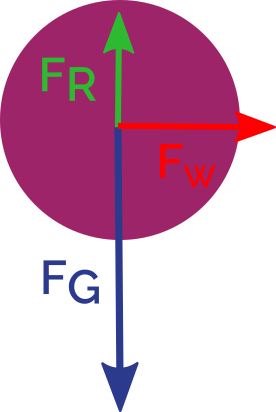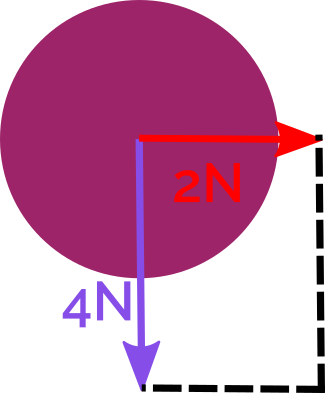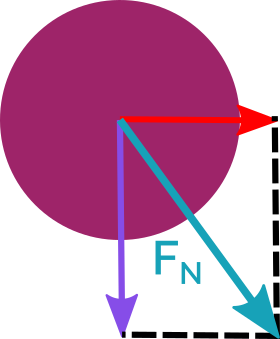# Force Calculator

Created by Julia Żuławińska
Reviewed by Steven Wooding
Last updated: Sep 28, 2022

Force calculator is here to help you calculate force from Newton's second law of motion. Read on to learn what force is, and what types of forces are there in classical mechanics. We'll also explain how to find force in exercises using the force formula. At the end, we also illustrate what net force is with an easy example.

If you need to find a specific force, maybe one of these calculators can help you:

## Force definition and the force equation

What is force?

🙋 Force is any interaction that, if unopposed, can change the motion of an object.

If asked, "What is force?" a non-physicist would probably think about pushing and pulling. A physicist would think about the change in the velocity of an object. To understand why let's look at the force equation:

$\footnotesize a = m / F$

where:

• $\footnotesize a$ is the acceleration of the object expressed in metre per second squared $\footnotesize[m/s^2]$;
• $\footnotesize m$ is the mass of an object in kilograms $\footnotesize [kg]$; and
• $\footnotesize F$ is the force measured in Newtons $\footnotesize [N]$.

Acceleration is the change of velocity over time. And, as you see from the force formula, the greater the force, the greater the acceleration. So, if something is speeding up, for example, a car, it can impart a significant force if it crashes on another car. This force is proportional to the car's mass and its (stopping) acceleration. Another example would be the human punch force, where the mass and acceleration of the body are directly proportional to the impact force.

To use the force calculator, input two of these variables: mass, acceleration, or force in any unit and get the missing number in a blink of an eye.

If you're calculating force on your own, always use the SI system to avoid mistakes. What is the SI unit of force? It's Newton $\footnotesize \bold{[N]}$ - named after Isaac Newton - mathematician, physicist, and discoverer of gravity. In SI base units, one Newton is equal to:

$\footnotesize 1N = 1 \space kg \cdot m/s^2$

To learn more about force units, go to our force converter.

## Newton's laws of motion

Newton came up with three laws of motion that explain the movement of all physical objects. They are the basics of all classical mechanics, which is also known as Newtonian mechanics.

1. Newton's first law of motion

An object will remain at rest or continue to move in uniform motion unless acted upon by an external force.

1. Newton's second law of motion

Force exerted by an object equals mass times acceleration of that object: $\footnotesize F = m \cdot a$.

1. Newton's third law of motion

When one body exerts a force on a second body, the second body exerts a force equal in magnitude and opposite in direction on the first body (for every action, there is always an equal but opposite reaction).

## Types of forces

All forces in are subjects to three of Newton's laws of motion.

• Gravitational force is the attraction between any two objects of nonzero mass. You walk on the ground instead of floating because of this force - gravity. It's exerted by everything around you, like the screen you're reading this on. It's just so small; it's unnoticeable.

• Normal force is the reaction to gravitational force - a perfect example of Newton's third law. When you're standing, you exert a force (equal to gravitational force) on the floor. The floor exerts on you a force of the same value.

• Friction is a force that opposes motion. It's proportional to the normal force acting between an object and the ground. In winter, you apply sand on icy surfaces to increase friction and prevent slipping.

• Tension is an axial force that passes through ropes, chains, springs, and other objects when there is external pulling. For example, if you're walking your dog, and it pulls you forward, it creates tension on its leash.

• Centrifugal force is a force acting on a rotating object. Have you ever been on a carousel? Do you remember the feeling of being pushed outwards? The centrifugal force caused that feeling.

• Pressure is the measure of the force applied on a surface. If you inflate a balloon, particles of air inside put pressure on the balloon. All particles feel the same force, so the balloon is inflated evenly.

## How to find force?

Let's look at a few exercises so that nothing can surprise you in your physics class.

1. Find the accelerating and retarding (stopping) force:

A cheetah has a mass of 50 kg. It accelerates from rest to 50 km/h in 3 seconds. Then it starts steadily slowing down and stops after 8 seconds.

• Accelerating force:

First, find acceleration:

$\footnotesize 50 \space \text{km/h}$ is equal to $\footnotesize 13.89 \space \text{m/s}$ (we calculated this with the speed converter).

Acceleration is equal to a difference in velocity over time:

$\footnotesize a = \frac{13.89\space \frac{m}{s} - 0}{ 3\space s} = 4.63 \space \frac{m}{s^2}$

Calculate accelerating force:

$\footnotesize F_a = m \cdot a = 50 \space kg \cdot 4.63 \space \frac{m}{s^2} = 231.5 \space N$

• Retarding force:

$\footnotesize a = \frac{0 - 13.89\space \frac{m}{s}}{ 8\space s} = -1.74 \space \frac{m}{s^2}$

$\footnotesize F_r = 50 \space kg \cdot (-1.74) \space \frac{m}{s^2} = -87 \space N$

Retarding force is negative, because it has an opposite direction to the accelerating force.

2. How much force do you need to accelerate an object ($\footnotesize \bold{ m = 2 \space kg}$) by $\footnotesize \bold{8 \frac{m}{s^2}}$? What about when the object is three times heavier? How does it affect the force?

• $\footnotesize F_1 = 2 \space kg \cdot 8 \space \frac{m}{s^2} = 16 \space N$

• $\footnotesize F_2 = 3 \cdot 2 \space kg \cdot 8 \space \frac{m}{s^2} = 48 \space N$

If the mass is three times heavier, the force needs to be three times bigger.

## What is net force?

Force is a vector. It means that it has a value and direction. That's why you can't add it like regular numbers (scalars).

The net force ($\footnotesize \text{F}_{\text{N}}$) is the sum of the vectors of all individual forces acting on an object. For example, let's look at a falling ball. It is affected by the gravitational force ($\footnotesize \text{F}_{\text{G}} = 5N$), air resistance ($\footnotesize \text{F}_R = 1 N$), and lateral force caused by wind ($\footnotesize \text{F}_W = 2 N$).1. First, find the net force of horizontal forces. They have an opposite direction so, in part, they cancel each other:
• $\footnotesize \text{F}_H = \text{F}_G - \text{F}_R = 5N - 1N = 4N$1. Now, find the net force of the two remaining forces.

Here, you can calculate it using Pythagorean theorem (in a right triangle: $\footnotesize a^2 + b^2 = c^2$). To learn more about adding vectors, go to the vector addition calculator.$\footnotesize \begin{split} F_H^2 + F_W^2 &= F_N^2 \\ 4^2 + 2^2 &= F_N^2 \\ 16 + 4 &= F_N^2 \\ F_N^2 &= 20 \\ F_N &= \sqrt{20} \\ F_N &= 2\sqrt 5 \end{split}$

The Net force acting on a ball equals 2√5 N.

Now that you know Newton's three laws of motion, and force definition, look at one of the calculators listed at the beginning. We explain there in detail all types of forces. We also recently did a fun experiment, where we tested what would win a race - a toilet paper or a bottle?. Check it out to learn something about mass moment of inertia, and acceleration!

## FAQ

### How to find acceleration with force and mass?

1. Divide force by mass.
2. Remember to use SI base units. That means, Newtons for force and kilograms for mass.
3. Enjoy your acceleration in meters per second squared.

### Is weight a force?

Weight is another name for gravitational force. In physics, mass and weight are not the same. Mass is a property of an object. It resists any change in motion. Weight is a force that acts on a mass because of gravity. On Earth, if your mass is 70 kilograms, your weight is about 700 Newtons (precisely 686.5 Newtons).

### Is force a vector?

Force is a vector. That means it's defined by both magnitude and direction. Scalars, like mass or length, are defined only by magnitude. So, if you apply two forces to an object, you can't add them like scalars. You need to take the direction into account and find the net force - the forces' vector sum.

### What is the formula for force?

The force formula is defined by Newton's second law of motion:

Force exerted by an object equals mass times acceleration of that object: F = m ⨉ a.

To use this formula, you need to use SI units: Newtons for force, kilograms for mass, and meters per second squared for acceleration.

### What happens when two forces act in the same direction?

When two forces act in the same direction, they add up and create a net force equal to their sum.

### How are force and motion related?

Force is any interaction that, if unopposed, can change the motion of an object. Without external force, a moving object will keep moving at a constant speed and direction, and an object at rest will stay at rest. If an external, unbalanced force is applied, an object will change the motion by changing its speed and/or direction.

The relationship between force and motion is defined by Newton's laws of motion.

### What are the types of forces?

Types of forces in physics:

• Contact forces - Normal force, applied force, frictional force, tension force, air resistance force; and
• Non-contact forces - Gravitational force, electrical force, magnetic force.

### Is acceleration a force?

Acceleration is not a force. Acceleration is the change of velocity over time. Same as force, acceleration is a vector, so it has both magnitude and direction. According to Newton's second law of motion, acceleration is proportionate to force: F = m ⨉ a. The direction of an object's acceleration is determined by the net force's direction acting on that object.

### Can net force be negative?

Net force can be both positive and negative. Net force is the sum of the vectors of all the individual forces that act on an object. Forces are always positive in magnitude, but, to make net force easier to calculate, we assume that forces aiming at opposite directions have different signs. Usually, we say that forces aiming to the right are positive, and to the left - negative. So, if you have two forces opposite in direction acting on an object and the net force aims to the left, you could say that the net force is negative.

### What is the difference between balanced and unbalanced forces?

Balanced forces have the same magnitude but opposite direction. They balance each other, and so cancel each other out. A classic example of balanced forces would be the gravitational force and the normal force acting on an object placed on a horizontal surface. Balanced forces cause no change in the direction or speed of an object.

Unbalanced forces don't have the same magnitude. If an unbalanced force acts on an object, it impacts the object's motion. For example, if something falls, it's because of gravitational force. Air resistance force counteracts gravity, but it doesn't balance it out if the object is too heavy.

### Do balanced forces cause a change in motion?

Balanced forces don't cause a change in motion. Two forces are balanced when they have the same magnitude and opposite direction, so they cancel each other out. Together, they don't affect the speed or direction of movement of an object.

### What is contact force?

Contact force is any force that needs contact to occur. Friction and normal force are both contact forces because they appear when an object is in contact with a surface. On the other hand, a non-contact force, like gravity, acts without contact with an object.

Julia ŻuławińskaMass (m)
kg
Acceleration (a)
m/s²
Force (F)
N
People also viewed…

### Body fat

Use the body fat calculator to estimate what percentage of your body weight comprises of body fat.

### Projectile motion experiment

Blast off with this super fun projectile motion experiment calculator! Create a simple launcher and learn the basics of projectile motion.

### Reynolds Number

The Reynolds number calculator will help you determine Reynolds number - an essential physical quantity in fluid mechanics.

### Titration

Use our titration calculator to determine the molarity of your solution.